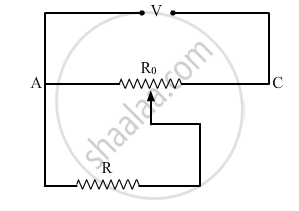# A Resistance of R Ω Draws Current from a Potentiometer as Shown in the Figure. the Potentiometer Has a Total Resistance Ro ω. a Voltage V is Supplied to the Potentiometer. Derive an Expression for the Voltage Across R When the Sliding Contact is in the Middle of the Potentiometer. - Physics

A resistance of R Ω draws current from a potentiometer as shown in the figure. The potentiometer has a total resistance Ro Ω. A voltage V is supplied to the potentiometer. Derive an expression for the voltage across R when the sliding contact is in the middle of the potentiometer.#### Solution

From the figure given in the question, current I through Ro is

I=V/R_o

Now, the sliding contact is in the middle of the wire. From the relation R=rhol/A

∝ l (where ρ and A are constants)

where l is the length of the wire, ρ is the resistivity and A is the area of the wire.

Resistance of half of the wire of the potentiometer is

R'=R_o/2

Now R' and R are in parallel; therefore, voltage across R and R' is equal. Therefore, voltage across R is

:.V'=I.R'=V/R_o"."R_o/2

=>V'=V/2

Concept: Metre Bridge
Is there an error in this question or solution?
2013-2014 (March) All India Set 2

Share